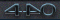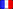Recherche

# Find The Total And Maximum In A Date Range With Numbers

jeudi 20 juin 2019, 15:00 , par MacMost
It is fairly easy to use a SUMIF function to find a total for rows that meet a condition. But there is no easy way to get the total for rows inside a range of dates. By using extra hidden columns, you can easily mark rows depending on whether they are in a date range, and then use SUMIF to get the total. Getting the maximum value in that range is a little trickier, but can be done with an additional column and the MAX function.

Check out Find The Total And Maximum In A Date Range With Numbers at YouTube for closed captioning and more options.
Video Transcript: Hi, this is Gary with MacMost.com. Let's look at finding the total, average, and maximum for a range of dated values in Numbers.
MacMost is brought to you by a community of supporters. Join us and get exclusive content at macmost.com/patreon.
So say we have a table here like this. It has a list of dates with a number after it. Sometimes the dates are repeated. Sometimes there is just one per date. Sometimes a date may be skipped. What we want to do is perform calculations on a range. For instance starting with June 3 and ending on June 7 get the total just of the rows that fall within those dates. Now if you were to use Sum If here it would be difficult because you don't want to see if it matches a certain date. You want to see if it becomes between a range of dates.
So what we're going to do is add a column here and that column is going to contain either true or false depending on whether the row is in this range. We'll start here with this first one and I'll hit the equals key to enter formula mode. I'll check first to see if this date is greater than or equal to the start date. So I'll click here and I'll do greater than or equal to >= and then this start date. I want to set this up to be an absolute value so it's always pointing to exactly the cell. Now what I get here is a true or false value depending upon whether or not 6/1 is on or after 6/3. Which, of course, is false here. If I were to copy and paste it further down you can see I get true here because 6/4 is after 6/3. That's a start.
But what I also want to do is to check and see whether or not it's before or on this date, less than or equal to <= this date. So I put a comma between them and I'm going to put an And function around the entire thing. So And with two parameters. One is is it >= to the start date. The other is <= to the end date. Make sure I set this one to be absolute as well.
Now when I copy this formula here and paste it in the entire row I get true and false values. You can see the true values here match the range of dates I specified here. If I were to change this and say have it start on 6/2 you could see it now includes 6/2. So now I can use Sum If to easily get the total. I'll do equals, Sum If and it will be this column here and whether it's true and then give me the sum of this column. I'll get that there. So I changed this, let's remove 2 and go back to 6/3, and you can see it updates. Name this column In Range?
Now it's just as easy to do average because I can do Average If. I can do the same thing here. I can do the test values, make it true, and then the values to average would be the ones in this column here and I get an average.
How about maximum? It would be great if there was a Max If function but there isn't. So we have to get really creative here. Would could try to create a range based on the first true and the last true or the first match of the date and the last match of the date. Then take that range and use that in a max formula to figure out what the maximum value is in that range. That would be really complex but possible.
However there's a much easier way to do it.
We're going to add another column here. This is going to be, I'll just call it Max Val. What I'm going to do is a simple formula equals the value here times the value here. True and false values are treated as either zero or one. So a false value would be zero and I get a value of zero there. I can copy and then paste throughout here and you can see I get zeroes everywhere that is outside of the date range and the actual value everywhere inside of the date range. So you would have to do a little more work here if you had negative values, say, but you're going to get zero for all the ones you don't want to count.
Now I can do a MAX formula here, select that entire row and I'll get a value here. In this case seventy which is the maximum there. If I were to cut off the range here and say only to the 4th you can see the maximum value now is \$65.00 on the 4th and it updates everything else. So now you have a way to change these two values here and then see different sums, averages, and the maximum value that falls in the date range.
One of the great things about doing it this way is it's not dependent on having these sorted. So it doesn't have to be in perfect date order. It doesn't care if you've got one in the middle here that doesn't match. So if I were to put this one as 6/1 you can see that changes to a false value here. It changes the sum there but it doesn't care that I've got this false value in the middle of all the true values.
Now I can select these two columns here and I can Hide them and pretend they're not there. These formulas here will still work to give me the amounts I want.
Related Posts:
How Do I Use Javascript To Get Values From a Numbers Spreadsheet? ― How To Do a Spreadsheet Sum Over At Changing Date Range? ― How Do I Capture Then Convert the Current Date/time In Numbers To a Static Date? ― How Do I Fill Numbers Cells With a Range Of Dates?
https://macmost.com/find-the-total-and-maximum-in-a-date-range-with-numbers.html
• Carpo• InsanelyGreatMac
• Mac Megasite
• Mac Merc
• Mac4Ever• Macs Only
• MacTouch• Power Page
• SVM Mac
• SVM Mac Blog• TidBITS
• TribuMac• xringsDate Actuelle
ven. 30 oct. - 15:02 CET